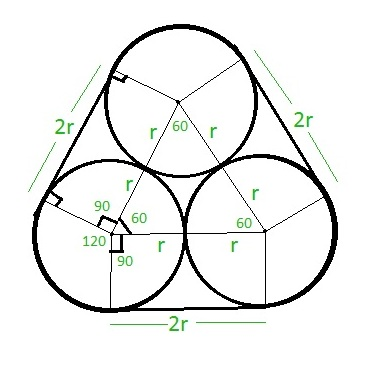# Length of rope tied around three equal circles touching each other

Given r is the radius of three equal circles touching each other. The task is to find the length of the rope tied around the circles as shown below:Examples:

Input: r = 7
Output: 86

Input: r = 14
Output: 172

## Recommended: Please try your approach on {IDE} first, before moving on to the solution.

Approach: As it can be clearly seen from above image, the part of the length of rope which is not touching the circle is 2r + 2r + 2r = 6r.
The part of the rope which is touching the circles make a sector of 120 degrees on each circle. Thus, three sectors of 120 degrees each can be considered as a complete one circle of 360 degrees.
Therefore, Length of rope touching the circle is 2 * PI * r where PI = 22 / 7 and r is the radius of the circle.
Hence, the total length of the rope will be ( 2 * PI * r ) + 6r.

Below is the implementation of the above approach:

## CPP

 `// C++ program to find the length ` `// of rope ` `#include ` `using` `namespace` `std; ` `#define PI 3.14159265 ` ` `  `// Function to find the length ` `// of rope ` `float` `length_rope( ``float` `r ) ` `{ ` `    ``return` `( ( 2 * PI * r ) + 6 * r ); ` `} ` ` `  `// Driver code ` `int` `main() ` `{ ` `    ``float` `r = 7; ` `    ``cout<<``ceil``(length_rope( r ))<

## C

 `// C program to find the length ` `// of rope ` `#include ` `#define PI 3.14159265 ` ` `  `// Function to find the length ` `// of rope ` `float` `length_rope( ``float` `r ) ` `{ ` `    ``return` `( ( 2 * PI * r ) + 6 * r ); ` `} ` ` `  `// Driver code ` `int` `main() ` `{ ` `    ``float` `r = 7; ` `    ``printf``(``"%f"``, ` `           ``length_rope( r )); ` `    ``return` `0; ` `} `

## Java

 `// Java code to find the length ` `// of rope ` `import` `java.lang.*; ` ` `  `class` `GFG { ` ` `  `    ``static` `double` `PI = ``3.14159265``; ` ` `  `    ``// Function to find the length ` `    ``// of rope ` `    ``public` `static` `double` `length_rope(``double` `r) ` `    ``{ ` `        ``return` `((``2` `* PI * r) + ``6` `* r); ` `    ``} ` ` `  `    ``// Driver code ` `    ``public` `static` `void` `main(String[] args) ` `    ``{ ` `        ``double` `r = ``7``; ` `        ``System.out.println(length_rope(r)); ` `    ``} ` `} `

## Python3

 `# Python3 code to find the length ` `# of rope ` `PI ``=` `3.14159265` `     `  `# Function to find the length ` `# of rope ` `def` `length_rope( r ): ` `    ``return` `( ( ``2` `*` `PI ``*` `r ) ``+` `6` `*` `r ) ` `     `  `# Driver code ` `r ``=` `7` `print``( length_rope( r )) `

## C#

 `// C# code to find the length ` `// of rope ` `using` `System; ` ` `  `class` `GFG { ` `    ``static` `double` `PI = 3.14159265; ` ` `  `    ``// Function to find the length ` `    ``// of rope ` `    ``public` `static` `double` `length_rope(``double` `r) ` `    ``{ ` `        ``return` `((2 * PI * r) + 6 * r); ` `    ``} ` ` `  `    ``// Driver code ` `    ``public` `static` `void` `Main() ` `    ``{ ` `        ``double` `r = 7.0; ` `        ``Console.Write(length_rope(r)); ` `    ``} ` `} `

## PHP

 ` `

Output:

```86
```

My Personal Notes arrow_drop_upCheck out this Author's contributed articles.

If you like GeeksforGeeks and would like to contribute, you can also write an article using contribute.geeksforgeeks.org or mail your article to contribute@geeksforgeeks.org. See your article appearing on the GeeksforGeeks main page and help other Geeks.

Please Improve this article if you find anything incorrect by clicking on the "Improve Article" button below.

Improved By : SURENDRA_GANGWAR